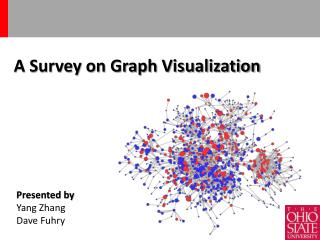DownloadDownload PresentationA Survey on Graph Visualization

# A Survey on Graph Visualization

Download Presentation## A Survey on Graph Visualization

- - - - - - - - - - - - - - - - - - - - - - - - - - - E N D - - - - - - - - - - - - - - - - - - - - - - - - - - -
##### Presentation Transcript

1. A Survey on Graph Visualization Presented by Yang Zhang Dave Fuhry

2. Challenges • Graph Layout • Make a concrete rendering of graph. • Scale for large graphs • Render on a computer screenwith limited pixels • High computational cost • Interaction • Show more detail for area of interest • Exploration for intuition

3. Force-Directed Layout • Nodes are modeled as physical bodies that are connected through springs (edges) • Pseudo code • Example • High running time • The typical force-directed algorithms are in general considered to have a running time equivalent to O(n3) , where n is the number of nodes of the input graph.

4. Pseudo Code set up initial node positions randomly loop total_kinetic_energy := 0 for each node net-force := (0, 0) for each other node net-force := net-force + repulsion( this_node, other_node ) for each spring connected to this node net-force := net-force + attraction( this_node, spring ) // without damping, it moves forever this_node.velocity := (this_node.velocity + timestep * net- force) * damping this_node.position := this_node.position + timestep * this_node.velocity total_kinetic_energy := total_kinetic_energy + this_node.mass *(this_node.velocity)^2  until total_kinetic_energy is less than some small number // the simulation has stopped moving

5. Zoom and pan • Zoom for graphs exact, adjustment of screen transformations • The internet map: http://internet-map.net/

6. Hierarchical Clustering • Successively applying the clustering process to clusters discovered by a previous step. • Can be navigated as tree

7. Example: ASK-GraphView

8. Dynamic Graphs Events in dynamic graphs

9. Event-View Interface

10. Event Visualization

11. Visualizing Cohesive Subgraphs • cohesive subgraphs • A subgraph in which vertices are densely connected. • Clique, K-Core, etc. • CSV • For each edge e, compute c(e), which is the size of the biggest clique that contains e. • Plot each vertex in the following order: • Randomly pick up the first vertex to plot; • Pick up the next vertex which shares an edge with maximum c(e) with previously plotted vertices. Plot the vertex with height = c(e) • The peaks indicate dense cohesive subgraphs

12. Directed K-Core : D-core • D = (V;E) is a digraph that is a set V of vertices and a set E of directed edges between them. • The min-in-degree and the min-out-degree of a digraph D are defined as • a (k; l)-D-core of D is a maximal sub-digraph F of D where • Denoted as

13. D-core matrix • For directed graph D, there is a unique (k; l)-D-core for each (k; l). We define D-core matrix AD as follows: AD(k; l) = size of (k; l)-D-core.

14. Tools Web-based tools • Cobweb: http://bioinformatics.charite.de/cobweb/ • Sigma.js: http://sigmajs.org/ • InfoVis: http://thejit.org/ • Cytoscape web: http://cytoscapeweb.cytoscape.org/ Applications • Gephi: http://gephi.org/ • Cytoscape: http://www.cytoscape.org/ • Guess: http://graphexploration.cond.org/index.html